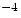Next: Data Records Up: Header Previous: Required Keywords

3.2.1.2 Random Parameter Reserved Keywords

The random parameters may be labeled and scaled in a fashion similar to the members and axes of a Basic FITS array, using the keywords PSCALn and PZEROn:

 physical_value = (FITS_value) × PSCALn + PZEROn (3.8)

The following keywords are reserved for describing random parameters.

• PTYPEn (character) is the name of the quantity represented by the nth random parameter.
• PSCALn (floating) gives the scale factor for random parameter n. If this keyword is absent, the scale factor is assumed to be 1.
• PZEROn (floating) gives the offset for random parameter n. If this keyword is absent, the offset is assumed to be zero.

Even though the random parameters must have the same data type as the array elements, greater precision can be achieved in the random parameters than in the data array through the use of repeated PTYPEn keywords. If more than one PTYPEn keyword has the same value, then the parameters associated with all those PTYPEn keywords are being used to represent the same quantity. The value of that quantity is derived by summing the true values derived for the parameters n corresponding to those PTYPEn keywords - the values derived using the stored values and the PSCALn and PZEROn values. For example, if

PTYPE1  = 'GLON    '
PTYPE2  = 'GLON    '
PSCAL1  =                  1.0
PSCAL2  =              1.0E-04

where GLON stands for Galactic longitude, then the value of Galactic longitude for each group is derived by adding the value of the first random parameter for that group to 10times the value of the second random parameter.

The rules governing units in random groups are the same as those for a primary data array, as given in section 3.1.1.4.Next: Data Records Up: Header Previous: Required Keywords